Space, shape and measurement: Calculate perimeter, surface area and volume in two- and three-dimensional geometrical shapes

# Unit 3: Total surface area of 3-D shapes

Natashia Bearam-Edmunds### Unit outcomes: Unit 3: Total surface area of 3-D shapes

By the end of this unit you will be able to:

• Calculate the surface area of the following geometric objects:
• Cubes
• Rectangular prisms
• Cylinders
• Triangular prisms
• Hexagonal prisms.
• Investigate the effect on the surface area of right prisms and cylinders, where one or more dimensions are multiplied by a constant factor k.

## What you should know

Before you start this unit, make sure you can:

• Find the area and perimeter of 2-D shapes. To revise area and perimeter review Unit 1 of this subject outcome.
• Find the volume of 3-D shapes. For more on volume review Unit 2 of the subject outcome.

## Introduction

Have you ever used far too much gift wrapping or not had enough to wrap a gift? If you work out the surface area of the object to be wrapped first, then you will know exactly how much gift wrapping you need. This is a simple example of how surface area can be useful, but there are many more important and practical uses for surface area both in everyday life and manufacturing.

Manufacturers of aluminium cans, for example, need to know how much material is needed to manufacture each can. They also need to calculate the surface area of each can to figure out how big the label for the can should be. If you were to paint the walls in your house, you would need to know what the surface area of the walls is so that you can buy the right amount of paint.

### Note

When you have access to an internet connection watch the video called “Surface Area in Real Life” to see another example of the use of surface area in real life.

Surface Area in Real Life (Duration: 15.47)## Surface area of right prisms and cylinders

To find out what surface area is, work through the following activity.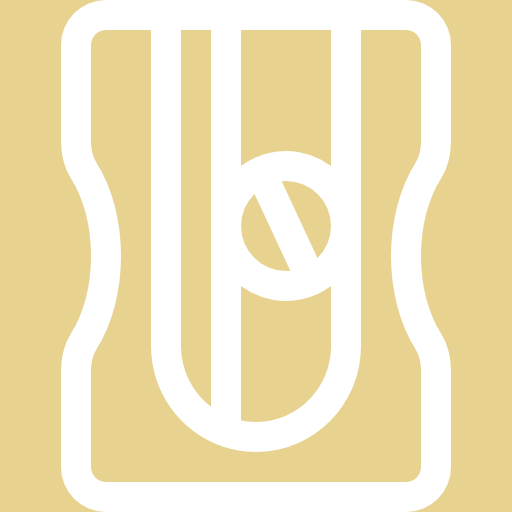### Activity 3.1: Explore surface area

Time required: 20 minutes

What you need:

• A pen and paper

What to do:

Look at this scenario and answer the questions that follow:

Thandi bought a gift for her mom and decided to put the gift into a box before wrapping it. She found an empty cardboard box, with square bases to use.1. How many faces does the box have?
2. Write down the shapes of all the faces and the number of each.
3. What name is given to this type of 3-D shape?
4. Thandi would like to wrap the box. What measurements does she need for each face?
5. Once she has the measurements what calculations will she need to do to cover all the aces?
6. If the box is unfolded, draw each of the faces that make up the box.
7. Thandi measured the box and found that the length and breadth are $\scriptsize \text{0}\text{.3 m}$ and the height is $\scriptsize 0.5\text{ m}$. What is the total surface area of the box?
8. If the gift has to go into a box with a surface area greater than its own, will this box work if the gift has a surface area of $\scriptsize 1\text{ }{{\text{m}}^{2}}$?

What did you find?

1. The box has $\scriptsize 6$ faces.
2. There are $\scriptsize 2$ square faces and $\scriptsize 4$ rectangular faces.
3. It is a rectangular prism.
4. To cover the box she would need to measure the length and breadth of each face.
5. To cover all the faces she must calculate the area of each face then add up the areas of each to get the total area.
6. A 3-D shape that is unfolded like this is called a net. When a prism is unfolded into a net, we can clearly see each of its faces. There is more than one way to unfold a 3-D solid into a net.
.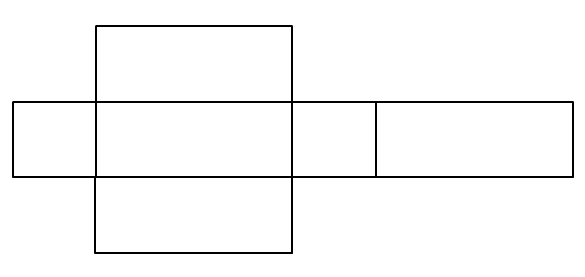### Note

For more on unfolding prisms into nets, you can watch the video called “Nets of polyhedra”.

Nets of polyhedra (Duration: 06.47)1. Let’s add in the measurements of each side of the net so we can find the total area..
$\scriptsize \begin{array}{l}\text{Total area}&=2\times \text{Area of square}+4\times \text{Area of rectangle}\\&=2(0.3\times 0.3)+4(0.5\times 0.3)\\&=0.78\text{ }{{\text{m}}^{2}}\end{array}$
2. The box will not work for the gift because its area ($\scriptsize 1\text{ }{{\text{m}}^{2}}$) is greater than the area of the box and we are told the area of the box must be greater than that of the gift.

The total surface area of a 3-D shape is the sum of the areas of all its faces (or exposed surfaces).

Below are more examples of right prisms and a cylinder that have been unfolded into nets.

A cube unfolded into a net is made up of six identical squares.

A triangular prism unfolded into a net is made up of two triangles and three rectangles. The sum of the widths of the rectangles is equal to the perimeter of the triangles.

A hexagonal prism unfolded into a net is made up of two hexagons and six rectangles.

### Note

If you have an internet connection, visit this interactive demonstration of the net of the hexagonal prism unfolding and to practise finding the surface area of a hexagonal prism.A cylinder unfolded into a net is made up of two identical circles and a rectangle with length equal to the circumference of the circles.### Example 3.1

Find the surface area of the following cylinder (correct to 1 decimal place).Solution

A cylinder unfolded into a net is made up of two identical circles and a rectangle with a length equal to the circumference of the circles.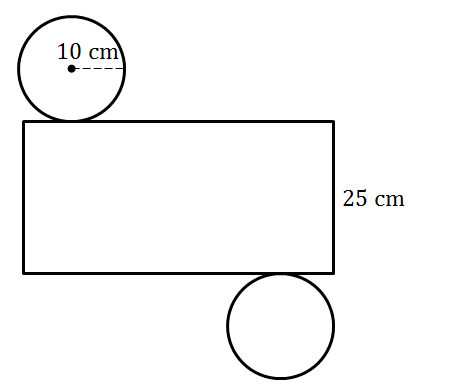Now we find the area of the different shapes of the net and add them together to find the total surface area.

$\scriptsize \begin{array}{l}\text{Area of rectangle}&=\text{circumference of circle}\times \text{length}\\&=2\pi r\times l\\&=2\pi (10)\times (25)\\&=500\pi \text{ c}{{\text{m}}^{2}}\end{array}$

$\scriptsize \begin{array}{l}\text{Area of circle}&=\pi {{r}^{2}}\\&=\pi {{(10)}^{2}}\\&=100\pi \text{ c}{{\text{m}}^{2}}\end{array}$

$\scriptsize \begin{array}{l}\text{Total surface area }&=\text{Area of rectangle }+2\text{(area of circle)}\\&=500\pi \text{ c}{{\text{m}}^{2}}+2(100\pi \text{ c}{{\text{m}}^{2}})\\&=500\pi \ \text{c}{{\text{m}}^{2}}+200\pi \ \text{c}{{\text{m}}^{2}}\\&=700\pi \text{ c}{{\text{m}}^{2}}\\&=2199.1\text{ c}{{\text{m}}^{2}}\end{array}$

The surface area of the cylinder is $\scriptsize 2199.1\text{ c}{{\text{m}}^{2}}$. Round off in the last step only.### Exercise 3.1

1. If a litre of paint covers an area of $\scriptsize 2\text{ }{{\text{m}}^{2}}$, how much paint (in whole litres) does a painter need for one coat of paint on the following objects:
1. A hexagonal table with base sides that are $\scriptsize 60\text{ cm}$ long and a perpendicular height of $\scriptsize 50\text{ cm}$. Round off to one decimal place.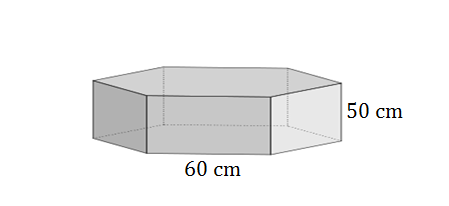2. A closed cylindrical water tank with a diameter of $\scriptsize 140\text{ cm}$ and a height of $\scriptsize \text{2}\text{.2 m}$. Round off to the nearest whole number.
(Hint: $\scriptsize 1\text{ }{{\text{m}}^{2}}=10\text{ }000\text{ c}{{\text{m}}^{2}}$)
2. Find the surface area of the following shape, which is made up of a rectangular prism with a cylinder on top. The rectangular prism is $\scriptsize \text{9 m}$ long, $\scriptsize \text{3 m}$ high and $\scriptsize \text{6 m}$ wide. The cylinder has a diameter of $\scriptsize \text{2 m}$ and its height is the same as the rectangular prism’s height. Round off to one decimal place.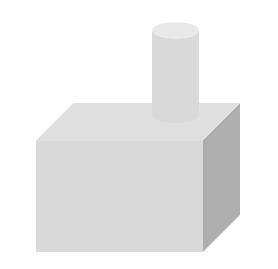The full solutions are at the end of the unit.

### Note

There is a formula we can use to easily find the total surface area of a closed right prism or cylinder.

$\scriptsize \text{TSA}=2\times \text{Area of the base}+\text{Perimeter of the base}\times \text{height}$

This formula works well for any closed 3-D prism or cylinder. But, for combined shapes and shapes that are open on the top or base you will need to modify the formula based on the question.### Example 3.2

Find the surface area of a fish tank that is the shape of a rectangular prism and is open on the top. The dimensions of the tank are $\scriptsize 5\text{ m}$ by $\scriptsize \text{3 m}$ by $\scriptsize 2.5\text{ m}$.

Solution

For this solution we will use the formula for total surface area.

$\scriptsize \text{TSA}=2\times \text{Area of the base}+\text{Perimeter of the base}\times \text{height}$

We are told that this tank is open on top so there is no need to multiply the area of the base by two as there is only one face with that measurement.

$\scriptsize \begin{array}{l}\text{TSA}&=\text{Area of the base}+\text{Perimeter of the base}\times \text{height}\\&=l\times b+2(l+b)h\\&=5\times 3+2(5+3)\times 2.5\\&=55\text{ }{{\text{m}}^{2}}\end{array}$

To practise using the formula to find total surface area do Activity 3.1 again.

## Multiplying dimensions by a constant factor

We saw in Unit 2 of this subject outcome that multiplying one or more of the dimensions of a prism or cylinder by a constant changed the volume. A change in dimensions will also affect the surface area of a 3-D shape. By understanding the relationship between the change in dimensions and its effect on surface area we can find simpler ways to calculate the new surface area of an object when its dimensions are scaled up or down.### Activity 3.2: Investigate the effect of scaling dimensions on surface area

Time required: 10 minutes

What you need:

• a pen and paper

What to do:

Consider a rectangular prism with dimensions $\scriptsize l,b,h$. Complete the table to see the effect on surface area when we multiply one, two and three of its dimensions by a constant factor of $\scriptsize 3$.

1. .
 Dimensions Surface area a) $\scriptsize l,b,h$ $\scriptsize \text{A}=2[(l\times h)+(l\times b)+(b\times h)]$ b) $\scriptsize l,b,3h$ $\scriptsize {{\text{A}}_{1}}=$ c) $\scriptsize l,3b,3h$ $\scriptsize {{\text{A}}_{2}}=$ d) $\scriptsize 3l,3b,3h$ $\scriptsize {{\text{A}}_{3}}=$ e) $\scriptsize kl,kb,kh$ $\scriptsize {{\text{A}}_{k}}=$
2. What effect does multiplying one dimension by a constant factor have on surface area?
3. What effect does multiplying two dimensions by a constant factor have on surface area?
4. If all three dimensions are multiplied by a constant factor of $\scriptsize k$ what is the effect on surface area?

What did you find?

1. .
 Dimensions Surface area a) $\scriptsize l,b,h$ $\scriptsize \begin{array}{l}\text{A}=2[(l\times h)+(l\times b)+(b\times h)]\\=2(lh+lb+bh)\end{array}$ b) $\scriptsize l,b,3h$ $\scriptsize \begin{array}{l}{{\text{A}}_{1}}=2[(l\times 3h)+(l\times b)+(b\times 3h)]\\=2(3lh+lb+3bh)\end{array}$ c) $\scriptsize l,3b,3h$ $\scriptsize \begin{array}{l}{{\text{A}}_{2}}=2[(l\times 3h)+(l\times 3b)+(3b\times 3h)]\\=2(3lh+3lb+{{3}^{2}}bh)\\=2\times 3(lh+lb+3h)\end{array}$ d) $\scriptsize 3l,3b,3h$ $\scriptsize \begin{array}{l}{{\text{A}}_{3}}=2[(3l\times 3h)+(3l\times 3b)+(3b\times 3h)]\\=2({{3}^{2}}lh+{{3}^{2}}lb+{{3}^{2}}bh)\\={{3}^{2}}\times 2(lh+lb+h)\\={{3}^{2}}\text{A}\end{array}$ e) $\scriptsize kl,kb,kh$ $\scriptsize \begin{array}{l}{{\text{A}}_{k}}=2[(kl\times kh)+(kl\times kb)+(kb\times kh)]\\=2({{k}^{2}}lh+{{k}^{2}}lb+{{k}^{2}}bh)\\={{k}^{2}}\times 2(lh+lb+h)\\={{k}^{2}}\text{A}\end{array}$
2. When one dimension is multiplied by a constant factor we cannot see a relationship between the original surface area and the new surface area.
3. When two dimensions are multiplied by a constant factor we cannot see a relationship between the original surface area and the new surface area.
4. When three dimensions are multiplied by a constant factor the new surface area is $\scriptsize {{k}^{2}}\times$ the original surface area.

We can use the relationship found between multiplying dimensions by a constant factor and the effect of surface area to easily calculate new surface areas without too much additional working out as shown in the next example.### Example 3.3

Consider a rectangular prism with a height of $\scriptsize 4\text{ cm}$ and base lengths of $\scriptsize \text{3 cm}$.

1. Calculate the surface area and volume.
2. Calculate the new surface area and volume if all dimensions are multiplied by a constant factor of $\scriptsize 5$.
3. Express the new surface area and volume as a factor of the original surface area and volume.

Solutions

1. Original surface area and volume:
$\scriptsize \begin{array}{l}\text{A}&=2\text{Area of base}+\text{Perimeter of base}\times h\\&=2(3\times 3)+2(3+3)\times 4\\&=66\text{ c}{{\text{m}}^{2}}\end{array}$
.
$\scriptsize \begin{array}{l}\text{V}&=\text{Area of base}\times \text{h}\\&=(3\times 3)\times 4\\&=36\text{ c}{{\text{m}}^{3}}\end{array}$
2. .
$\scriptsize \begin{array}{l}{{\text{A}}_{{\text{new}}}}&={{5}^{2}}\text{A}\\&\text{=25}\times \text{66}\\&\text{=1}\ \text{650 c}{{\text{m}}^{2}}\end{array}$
.
$\scriptsize \begin{array}{l}{{\text{V}}_{{\text{new}}}}&={{5}^{3}}\text{V}\\&=125\times 1\ 650\\&=206\text{ }250\text{ c}{{\text{m}}^{3}}\end{array}$
3. .
$\scriptsize \begin{array}{l}\displaystyle \frac{{{{\text{A}}_{{\text{new}}}}}}{\text{A}}&=\displaystyle \frac{{1\ 650}}{{66}}\\&=25\\\therefore {{\text{A}}_{{\text{new}}}}&={{5}^{2}}\text{A}\end{array}$
.
$\scriptsize \begin{array}{l}\displaystyle \frac{{{{\text{V}}_{{\text{new}}}}}}{\text{V}}&=\displaystyle \frac{{206\text{ }250}}{{1\text{ }650}}\\&=125\\\therefore {{\text{V}}_{{\text{new}}}}&={{5}^{3}}\text{V}\end{array}$### Exercise 3.2

1. Given a prism with surface area of $\scriptsize 2\text{ }520\text{ }{{\text{m}}^{2}}$, calculate the new surface area if all dimensions are tripled.
2. Consider a cylinder with a radius of $\scriptsize r$ and a height of $\scriptsize h$. Calculate the surface area (in terms of $\scriptsize r$ and $\scriptsize h$) if the radius is multiplied by a constant factor of $\scriptsize k$.

The full solutions are at the end of the unit.

## Summary

In this unit you have learnt the following:

• How to find the surface area of right prisms and cylinders.
• How to draw the nets of right prisms and cylinders to calculate the surface area.
• How to use the surface area formula.
• How to find the new surface area when dimensions are multiplied by a constant factor.

# Unit 3: Assessment

#### Suggested time to complete: 20 minutes

1. Here we look at Alfred’s ‘piggy bank’ again. Alfred’s ‘piggy bank’ is cylindrical in shape, is constructed from tin and has a slot at the top. Remember that the area of the slot is $\scriptsize 2\text{ c}{{\text{m}}^{2}}$, the diameter of the base of the ‘piggy bank’ is $\scriptsize 11\text{ cm}$ and the height of the ‘piggy bank’ is $\scriptsize 7\text{ cm}$.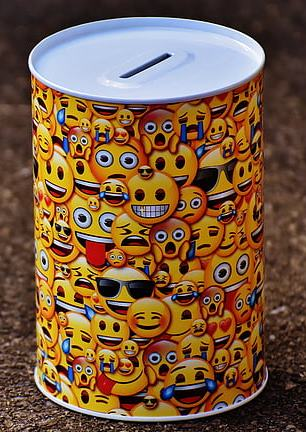.
Calculate the surface area of material that was used to manufacture this ‘piggy bank’.
2. If the length of each side of a hexagonal prism is quadrupled, what will the surface area of the new prism be?

The full solutions are at the end of the unit.

# Unit 3: Solutions

### Exercise 3.1

1. .
1. We need to find the surface area of the hexagonal table to find out how much paint is needed to paint it. A hexagonal prism is made up of two hexagons and six rectangles.
$\scriptsize \begin{array}{l}\text{Surface area}&=2\text{(Area of hexagon)}+6\text{(Area of rectangle)}\\&=2(\displaystyle \frac{{3\sqrt{3}}}{2}{{s}^{2}})+6(l\times b)\text{ The breadth of the rectangles is the same as the height of the prism}\\&=3\sqrt{3}{{(60)}^{2}}+6(60\times 50)\\&=36~706.1\text{ c}{{\text{m}}^{\text{2}}}\end{array}$
Convert from $\scriptsize \text{c}{{\text{m}}^{\text{2}}}$ to $\scriptsize {{\text{m}}^{2}}$.
$\scriptsize \begin{array}{l}1\text{ }{{\text{m}}^{2}}&=10\text{ }000\text{ c}{{\text{m}}^{2}}\\36\text{ }706.1\text{ c}{{\text{m}}^{\text{2}}}&=\displaystyle \frac{{36\text{ }706.1}}{{10\text{ }000}}\text{ }{{\text{m}}^{\text{2}}}\\&=3.67061\text{ }{{\text{m}}^{\text{2}}}\end{array}$
.
Therefore, the painter would need $\scriptsize 2$ litres of paint for one coat of the table since $\scriptsize 2$ litres of paint would cover an area of $\scriptsize \text{4 }{{\text{m}}^{2}}$, which is more than the area of the table.
2. The diameter of the tank is $\scriptsize 140\text{ cm}$ so the radius is $\scriptsize 70\text{ cm}$. Remember to use the same units throughout. Therefore, convert $\scriptsize 70\text{ cm}$ to $\scriptsize 0.7\text{ m}$. The height $\scriptsize \text{2}\text{.2 m}$.
$\scriptsize \begin{array}{l}\text{Area of rectangle}&=\text{circumference of circle}\times \text{length}\\&=2\pi r\times l\\&=2\pi (0.7)\times (2.2)\\&=\displaystyle \frac{{77}}{{25}}\pi \text{ }{{\text{m}}^{2}}\end{array}$
$\scriptsize \begin{array}{l}\text{Area of circle}&=\pi {{r}^{2}}\\&=\pi {{(2.2)}^{2}}\\&=\displaystyle \frac{{121}}{{25}}\pi \text{ }{{\text{m}}^{2}}\end{array}$
$\scriptsize \begin{array}{l}\text{Total surface area }&=\text{Area of rectangle }+2\text{(area of circle)}\\&=\displaystyle \frac{{77}}{{25}}\pi \text{ }{{\text{m}}^{2}}+2(\displaystyle \frac{{121}}{{25}}\pi \text{ }{{\text{m}}^{2}})\\&=\displaystyle \frac{{319}}{{25}}\pi \text{ }{{\text{m}}^{2}}\\&=40.09\text{ }{{\text{m}}^{2}}\end{array}$
The painter would need to cover $\scriptsize \displaystyle \frac{{40}}{2}=20\text{ }{{\text{m}}^{2}}$.
$\scriptsize 1$ litre of paint would cover an area of $\scriptsize \text{2 }{{\text{m}}^{2}}$, so he therefore needs $\scriptsize 10$ litres of paint.
2. The surface area by definition only takes into account exposed surfaces. So, in this question we must not include two circles when we calculate the surface area of the cylinder as only the top circle is exposed.
$\scriptsize \begin{array}{l}\text{Total surface are}{{\text{a}}_{{\text{cylinder}}}}\text{ }&=\text{Area of rectangle }+\text{area of circle}\\&=2\pi rl+\pi {{r}^{2}}\text{ }\\&=2\pi (1)(3)+\pi {{(1)}^{2}}\\&=7\pi \text{ }{{\text{m}}^{2}}\end{array}$
.
We must also exclude the base circle of the cylinder from surface area of the rectangular prism. To do that, we must remember that the section where the cylinder meets the top face of the prism will not be exposed. So, we must subtract the area of the circle from the area of the top rectangular face to find the rectangular prism’s surface area..
$\scriptsize \begin{array}{l}\text{Total surface are}{{\text{a}}_{{\text{rectangular prism}}}}&=(9\times 6-\pi {{r}^{2}})+(9\times 6)+2(9\times 3)+2(6\times 3)\\&=54-\pi {{(1)}^{2}}+54+54+36\\&=(198-\pi )\text{ }{{\text{m}}^{2}}\end{array}$.
.
For total surface area of the combined shape add the surface area of the cylinder to the surface area of the rectangular prism, and exclude the area of the circular base twice.
.
$\scriptsize \begin{array}{l}\text{Total surface area}&=2\pi rl+\pi {{r}^{2}}+(198-\pi )\text{ }\\&=2\pi (1)(3)+\pi {{(1)}^{2}}+(198-\pi )\text{ }\\&\text{=8}\pi +\pi +198-\pi \\&=223\text{ }{{\text{m}}^{2}}\end{array}$

Back to Exercise 3.1

### Exercise 3.2

1. .
$\scriptsize \begin{array}{l}{{\text{A}}_{{\text{new}}}}&={{3}^{2}}(2\text{ }520)\\&=22\text{ }680\text{ }{{\text{m}}^{2}}\end{array}$
2. .
$\scriptsize \begin{array}{l}\text{Surface Area}&=2(\pi {{r}^{2}})+2\pi rh\\\text{Surface Area new}&=2\pi {{(kr)}^{2}}+2\pi krh\\&=2(\pi {{r}^{2}})\cdot {{k}^{2}}+2\pi rh\cdot k\\&=k(2\pi {{r}^{2}}k+2\pi rh)\end{array}$

Back to Exercise 3.2

### Unit 3: Assessment

1. The area of the slot of $\scriptsize 2\text{ c}{{\text{m}}^{2}}$ must be subtracted from the area of the top circle since that is not covered in tin. The diameter of the base of the ‘piggy bank’ is $\scriptsize 11\text{ cm}$ so the radius is $\scriptsize \text{5}\text{.5 cm}$ and the height of the ‘piggy bank’ is $\scriptsize 7\text{ cm}$.
.
$\scriptsize \begin{array}{l}\text{Area}&=\pi {{r}^{2}}+(\pi {{r}^{2}}-2)+2\pi rh\\&=2\pi {{r}^{2}}-2+2\pi rh\\&=2\pi {{(5.5)}^{2}}-2+2\pi (5.5)(7)\\&=429.97\text{ c}{{\text{m}}^{2}}\end{array}$
2. .
$\scriptsize \begin{array}{l}\text{Surface area}&=2\text{(Area of hexagon)}+6\text{(Area of rectangle)}\\&=2(\displaystyle \frac{{3\sqrt{3}}}{2}{{s}^{2}})+6(l\times b)\\&\text{= }3\sqrt{3}{{s}^{2}}+6lb\end{array}$
.
$\scriptsize \begin{array}{l}\text{Surface are}{{\text{a}}_{{\text{new}}}}&\text{= }3\sqrt{3}{{(4s)}^{2}}+6(4l)(4b)\\&=3\sqrt{3}(16{{s}^{2}})+6(16lb)\\&=16(3\sqrt{3}{{s}^{2}}+6lb)\\&={{4}^{2}}\text{ original surface area}\end{array}$

Back to Unit 3: Assessment